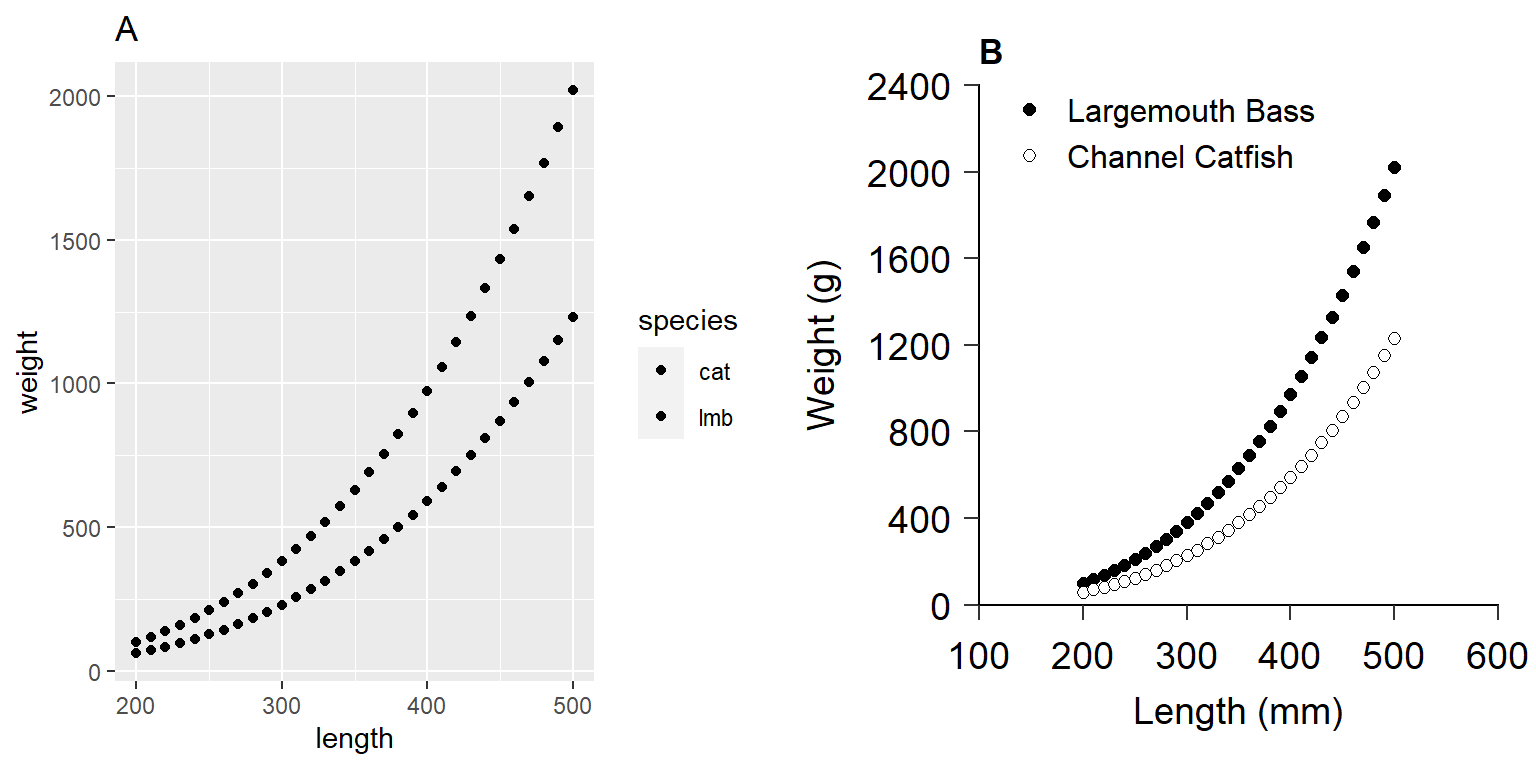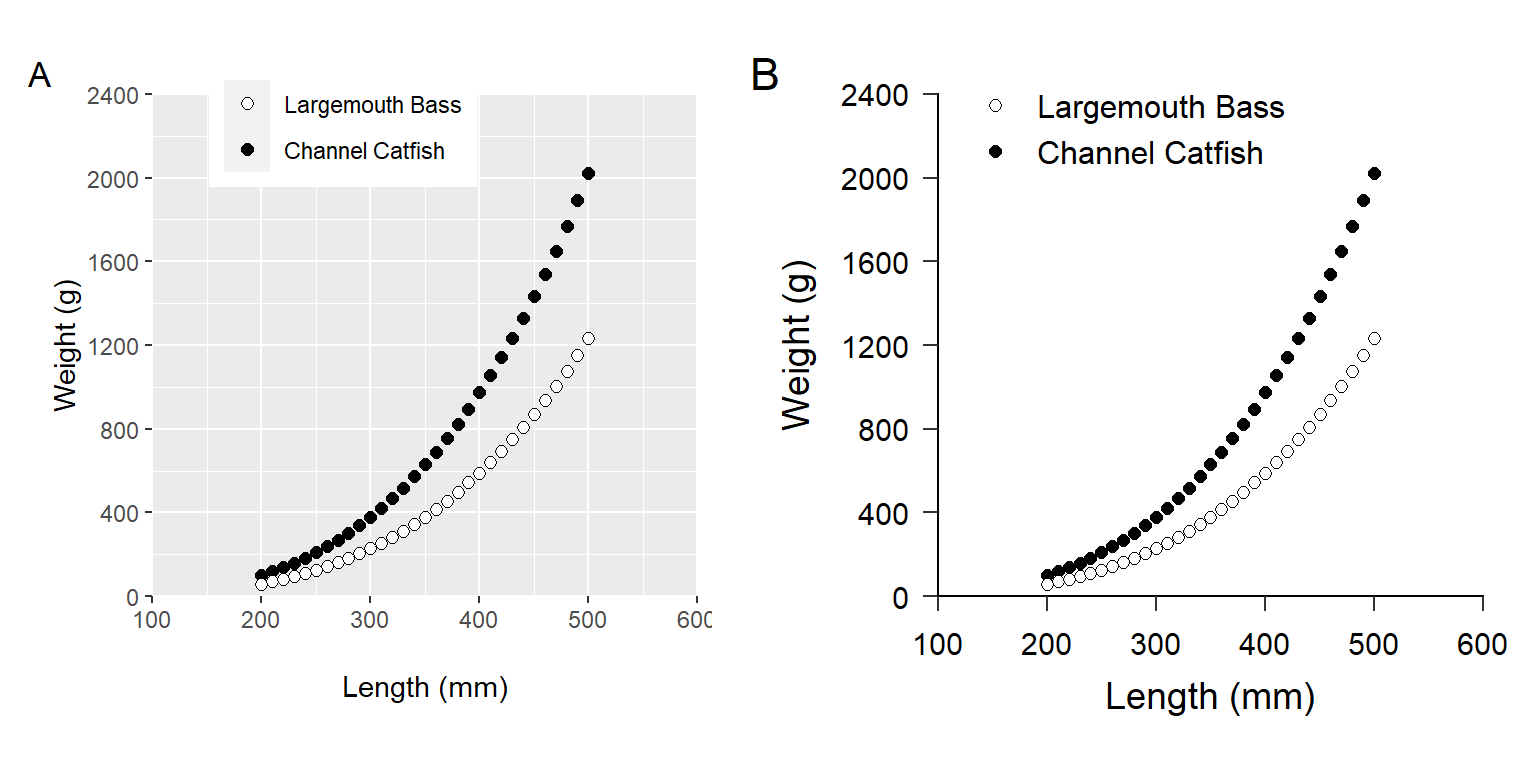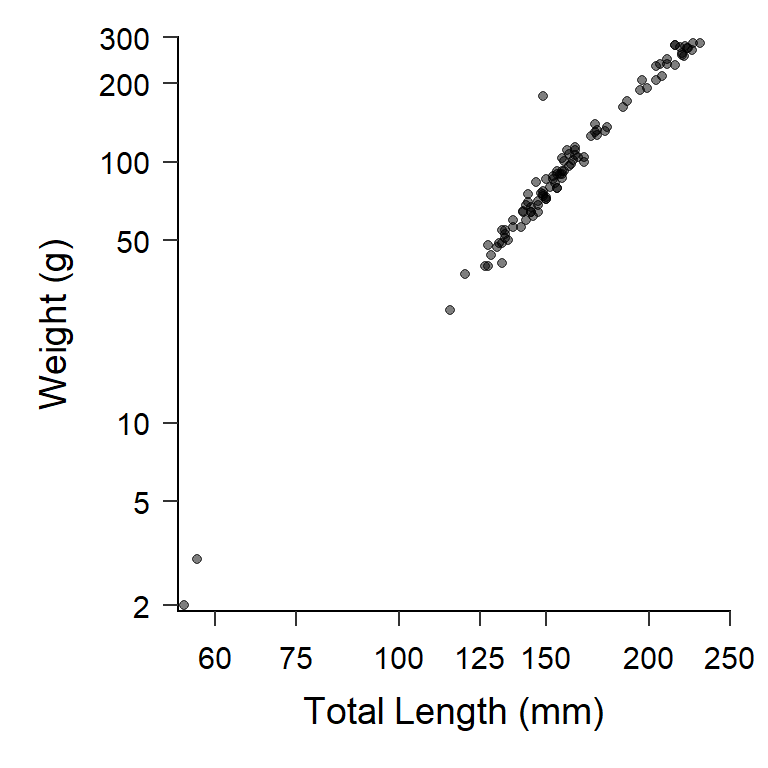# AFS Style in ggplot2 Figures

Suggestions for the Glassic et al. (2019) process for modifying ggplot2 graphs to match the AFS Style Guide.
ggplot2
Author

Derek H. Ogle

Published

Dec 22, 2022

Modified

Feb 13, 2023

Note

The following packages are loaded for use below.

``````library(ggplot2)
library(patchwork)  # for positioning multiple plots``````

# Introduction

Glassic et al. (2019) showed how to modify default `ggplot2` graphics to meet American Fisheries Society (AFS) Style Guide requirements.1 They provided an example with accompanying script to help users implement their suggestions.2 Since their publication, `ggplot2` has been updated, which did not break their code but leads to some warnings of changed arguments. In this post, I update their script and provide some suggestions that I think will allow their recommendations to be more easily implemented.

• 1 They also did the same for base R graphics.

• 2 The `ggplot2` script is available here.

• The first part of their script3 creates a data frame called `length_weight_data` with simple weight and length data for two species (`lmb` and `cat`).

• 3 Which is not shown here as I want to focus on graphing.

• ``````# a quick peek at the data
FSA::peek(length_weight_data,n=6)``````
``````#R|     species length    weight
#R|  1      lmb    200  100.7561
#R|  12     lmb    310  422.8894
#R|  25     lmb    440 1330.5176
#R|  37     cat    250  125.5493
#R|  50     cat    380  498.6630
#R|  62     cat    500 1231.4228``````

# Glassic et al. (2019) Figures

Glassic et al. (2019) first demonstrated a default `ggplot2` plot (Figure 1 (A)).4

• 4 This is their code exactly, except that I added indentations for easier reading.

• ``````len_wt_default <- ggplot(data = length_weight_data,
aes(x = length, y = weight, fill = species)) +
geom_point() +
labs(title = "A")``````

They then modified this plot to fit the AFS Style Guide requirements (Figure 1 (B)).5

• 5 I modified their `legend.position=` slightly due to website size constraints.

• ``````len_wt_afs <- ggplot(data = length_weight_data,
aes(x = length, y = weight, fill = species)) +
#set symbol shape and size
geom_point(shape = 21, size = 2) +
#set the limits and tick breaks for the y-axis
scale_y_continuous (limits = c(0,2400), expand = c(0,0),
breaks = seq(0,2400,400)) +
#set the limits and tick spacing for the x-axis
scale_x_continuous(limits = c(100,600), expand = c(0,0),
breaks = seq(100,600,100)) +
#adjust the order of the legend, make new labels, and select the symbol colors
scale_fill_manual(limits = c("lmb", "cat"),
labels = c("Largemouth Bass", "Channel Catfish"),
values = c("black", "white")) +
ggtitle ("B") +
#label the y-axis
ylab("Weight (g)") +
#label the x-axis
xlab("Length (mm)") +
#add legend title, but left blank here because we want a legend but no title
labs(fill = "") +
#makes the figure background white without grid lines
theme_classic() +
#below are theme settings that provide unlimited control of your figure
#and can be a template for other figures set the size, spacing, and color
#for the y-axis and x-axis titles
theme(axis.title.y = element_text(size = 14,
margin = margin(t = 0, r = 10, b = 0, l = 0),
colour = "black"),
axis.title.x = element_text(size = 14,
margin = margin(t = 10, r = 0, b = 0, l = 0),
colour = "black"),
#set the font type
text = element_text(family = "Times New Roman"),
#modify plot title, the B in this case
plot.title = element_text(face = "bold", family = "Arial"),
#position the legend on the figure
legend.position = c(0.35,0.95),
#adjust size of text for legend
legend.text = element_text(size = 12),
#margin for the plot
plot.margin = unit(c(0.5, 0.5, 0.5, 0.5), "cm"),
#set size of the tick marks for y-axis
axis.ticks.y = element_line(size = 0.5),
#set size of the tick marks for x-axis
axis.ticks.x = element_line(size = 0.5),
#adjust length of the tick marks
axis.ticks.length = unit(0.2,"cm"),
#set size and location of the tick labels for the y axis
axis.text.y = element_text(colour = "black", size = 14, angle = 0,
vjust = 0.5, hjust = 1,
margin = margin(t = 0, r = 5, b = 0, l = 0)),
#set size and location of the tick labels for the x axis
axis.text.x = element_text(colour = "black", size = 14, angle = 0,
vjust = 0, hjust = 0.5,
margin = margin(t = 5, r = 0, b = 0, l = 0)),
#set the axis size, color, and end shape
axis.line = element_line(colour = "black", size = 0.5, lineend = "square"))``````

They then arranged the two plots side-by-side.6

• 6 I used `gridExtra::` here so as not to load the entire package.

• ``````ggplot_figure <- gridExtra::grid.arrange(len_wt_default, len_wt_afs, ncol = 2)
ggplot_figure``````Figure 1: Figures made with ggplot2 including one with (A) default values and (B) a custom figure that adheres to American Fisheries Society guidelines for authors. This reproduces Figure 2 in Glassic et al. (2019).

Finally, Glassic et al. (2019) demonstrated how to save the plot with a custom width, height (holding a specific aspect ratio), and resolution.

``````ggsave(ggplot_figure, file = "ggplot_figure.tiff",
width = 20.32, height = 7.62, units = "cm", dpi = 300)``````

# Issues that Must be Addressed

More recent versions of `ggplot2` use `linewidth=` rather than `size=` in all line-related elements. This needs to be changed in the authors’ use of `axis.tick.y=`, `axis.tick.x=`, and `axis.line=`.

When I first ran the author’s code I received an error about a missing font. I followed the advice in this guide from the `extrafont` package, which mostly fixed the issue.7

• 7 I still receive a font-based warning when rendering the Quarto document.

•

I separate the construction of the plot by Glassic et al. (2019) into three parts. The first part consists of items that are specific to the plot and that cannot be set globally for use in other plots. These are items likes `data=`, `aes()`, `geom()`s, `scale()`s, and labels. The second part consists of items that will likely need to be held constant across different plots. These are items like font size, tick lengths, margins, etc. These are largely the items that Glassic et al. (2019) adjust with `theme()`. THe third part is positioning two plots side-by-side as one overall figure.

Below I explain some suggested modifications to the plot specific items. I then show how to move most of the elements that will be common across plots into a custom theme that can be easily applied to any plot. Finally, I demonstrate using `patchwork` rather than `gridExtra` to place plots next to each other.

## Plot-Specific Elements

I suggest the following changes to the plot-specific elements. Implementing these suggestions will result in Figure 2 (A).

• Name the second argument to `ggplot()` (i.e., `mapping=`). `ggplot()` is smart enough to figure this out but I think it is awkward to name the first argument (i.e., `data=`) but not the second.
• I moved the text for the axis labels (i.e., titles) into `scale_y_continuous()` and `scale_x_continuous()` for simplicity (and thus removed `ylab()` and `xlab()`).
• I changed `expand=c(0,0)` in `scale_y_continuous()` and `scale_x_continuous()` to `expand=expansion(mult=c(0,0))` to follow more recent `ggplot2` conventions. This still results in no scale expansion at the top or bottom of axis.
• I removed `limits=` in `scale_fill_manual()` and used a named vector in `values=` to accomplish the same task. I think this makes it easier to see which category gets which color.
• I removed `ggtitle()` as I am going to accomplish that same task with `patchwork` as shown below (i.e., where the two plots are place side-by-side).
• I used `legend.title=element_blank()` in `theme()` rather than `labs(fill="")` to remove to legend title. I think(?) this handles the freed up space better.
• I included `legend.position=` in `theme()` here because Glassic et al. (2019) positioned the legend within the plot area and, thus, will need to be set specific to each plot (i.e., will need to manually find a “white area”).
``````len_wt_afs1 <- ggplot(data=length_weight_data,
mapping=aes(x=length,y=weight,fill=species)) +
# set symbol shape and size
geom_point(shape=21,size=2) +
# set the limits, tick breaks, and scale expansion for the y- and x-axis
scale_y_continuous(name="Weight (g)",
limits=c(0,2400),breaks=seq(0,2400,400),
expand=expansion(mult=c(0,0))) +
scale_x_continuous(name="Length (mm)",limits=c(100,600),breaks=seq(100,600,100),
expand=expansion(mult=c(0,0))) +
# set the symbol colors and make new labels for each level
scale_fill_manual(values=c("lmb"="black","cat"="white"),
labels=c("Largemouth Bass","Channel Catfish")) +
theme(
# remove legend title
legend.title=element_blank(),
# set legend position within the plot
legend.position = c(0.35,0.95)
)``````

## Custom Theme for All Plots

Theme elements that will be consistent across plots can be put into a custom theme that can then be easily applied to any plot. The code below, for example, shows the start of a new theme called `theme_AFS()` that has `theme_classic()` as its base but will have several elements replaced. `theme_AFS()` will use a base font size of 14 and Times New Roman as defaults, which will minimize some of the authors’ code (as described below).

``````theme_AFS <- function(base_size=14,base_family="Times New Roman") {
theme_classic(base_size=base_size,base_family=base_family) +
theme(
### Change theme elements here ###
)
}``````

Within `theme()` I add nearly all of the elements that Glassic et al. (2019) included, though I reordered the items in a way that makes sense to me (e.g., axis title then axis tick labels then axis ticks then axis line). Other adjustments are:

• I removed `text=` because the font family was already set with `base_family=`.
• I removed `legend.position=` because, as described above, Glassic et al. (2019) positioned the legend within the plot area.
• I removed `size=14` from `axis.title.y=`, `axis.title.x=`, `axis.text.y=`, and `axis.text.x=` because that was set with `base_size=`.
• I replaced `axis.ticks.y=` and `axis.ticks.x=` with `axis.ticks=` because their elements were the same and using `axis.ticks=` will set both at the same time.
``````theme_AFS <- function(base_size=14,base_family="Times New Roman") {
theme_classic(base_size=base_size,base_family=base_family) +
theme(
# modify plot title,the B in this case
plot.title=element_text(family="Arial",face="bold"),
# margin for the plot
plot.margin=unit(c(0.5,0.5,0.5,0.5),"cm"),
# set axis label (i.e., title) colors and margins
axis.title.y=element_text(colour="black",margin=margin(t=0,r=10,b=0,l=0)),
axis.title.x=element_text(colour="black",margin=margin(t=10,r=0,b=0,l=0)),
# set tick label color, margin, and position and orientation
axis.text.y=element_text(colour="black",margin=margin(t=0,r=5,b=0,l=0),
vjust=0.5,hjust=1),
axis.text.x=element_text(colour="black",margin=margin(t=5,r=0,b=0,l=0),
vjust=0,hjust=0.5,),
# set size of the tick marks for y- and x-axis
axis.ticks=element_line(linewidth=0.5),
# adjust length of the tick marks
axis.ticks.length=unit(0.2,"cm"),
# set the axis size,color,and end shape
axis.line=element_line(colour="black",linewidth=0.5,lineend="square"),
# adjust size of text for legend
legend.text=element_text(size=12)
)
}``````

`theme_AFS()` can then be “added” to any plot to apply its elements. For example, applying `theme_AFS()` to the code that produced Figure 2 (A) will produce Figure 2 (B). It is important, however, to make sure that the plot-specific `theme()` elements are applied after the custom theme.

``````len_wt_afs2 <- ggplot(data=length_weight_data,
mapping=aes(x=length,y=weight,fill=species)) +
# set symbol shape and size
geom_point(shape=21,size=2) +
# set the limits, tick breaks, and scale expansion for the y- and x-axis
scale_y_continuous(name="Weight (g)",
limits=c(0,2400),breaks=seq(0,2400,400),
expand=expansion(mult=c(0,0))) +
scale_x_continuous(name="Length (mm)",
limits=c(100,600),breaks=seq(100,600,100),
expand=expansion(mult=c(0,0))) +
# set the symbol colors and make new labels for each level
scale_fill_manual(values=c("lmb"="black","cat"="white"),
labels=c("Largemouth Bass","Channel Catfish")) +
theme_AFS() +
theme(
# remove legend title
legend.title=element_blank(),
# set legend position within the plot
legend.position = c(0.35,0.95)
)``````
Hint

See the “Themes” chapter of Wickham et al. (2022) for an excellent description of using themes in `ggplot2` graphics.

## Using `patchwork` to Position Plots

The `patchwork` package provides simple but extensive tools for combining multiple plots. Two plots can simply be “added” together to place them side-by-side (Figure 2). `plot_annotation()` is used to add letters to the two panels.8

• 8 See the excellent documentation for `patchwork` to better understand what this package can do.

• ``````len_wt_afs1 + len_wt_afs2 +
plot_annotation(tag_levels="A")``````Figure 2: Figures made with ggplot2 including one with (A) only plot-specific modifications to default values and (B) with plot specific modifications and a custom theme that adheres to American Fisheries Society guidelines for authors. Panel B reproduces panel B in Figure 1 above and Figure 2 in Glassic et al. (2019).
Hint

Annotating plots with `patchwork` will place the annotations on the very edge of the figure panel. Use `ggtitle()` to move them more to the right as Glassic et al. (2019) had them.

# Conclusion

Glassic et al. (2019) provided an excellent example and script for how to use `ggplot2` in R to make figures that adhere to the AFS Style Guidelines. Here I provide some suggestions to update their script for recent changes to `ggplot2`. More importantly their suggestions were put into a custom theme that can be easily applied to other figures to ensure consistency (and adherence to the AFS Style Guide). As an example, `theme_AFS()` is applied to a `ggplot2` length frequency histogram below (Figure 3).9

• 9 This uses the `BluegillLM` data from the `FSAdata` package; thus, you must have `FSAdata` installed.

• ``````data(BluegillLM,package="FSAdata")
ggplot(data=BluegillLM,mapping=aes(x=tl)) +
geom_histogram(binwidth=10,boundary=0,color="black",fill="gray25") +
scale_y_continuous(name="Number of Bluegill",
limits=c(0,25),breaks=seq(0,25,5),
expand=expansion(mult=c(0,0))) +
scale_x_continuous(name="Total Length (mm)",
limits=c(0,250),breaks=seq(0,250,25),
expand=expansion(mult=c(0,0))) +
theme_AFS()``````
Hint

This post describes how to make length frequency histograms in `ggplot2`.

Moreover, if you are working on a project where you will use `theme_AFS()` for all figures then you can set it as the default theme at the beginning of your script with `theme_set()`.

``theme_set(theme_AFS())``

With this, Figure 4 automatically has `theme_AFS()` applied.

``````ggplot(data=BluegillLM,mapping=aes(x=tl,y=wght)) +
geom_point(alpha=0.5) +
scale_y_continuous(name="Weight (g)",
limits=c(NA,300),breaks=c(2,5,10,50,100,200,300),
expand=expansion(mult=c(0.01,0)),
trans="log10") +
scale_x_continuous(name="Total Length (mm)",
limits=c(NA,250),breaks=c(60,75,100,125,150,200,250),
expand=expansion(mult=c(0.01,0)),
trans="log10")``````Figure 4: Weight-length relationship for Lake Mary Bluegill. Note that both axes are on the log scale.

## References

Glassic, H. C., K. C. Heim, and C. S. Guy. 2019. Creating figures in r that meet the afs style guide: Standardization and supporting script. Fisheries 44:539–544.
Wickham, H., D. Navarro, and T. L. Pedersen. 2022. ggplot2: Elegant Graphics for Data AnalysisThird. Springer.

## Citation

BibTeX citation:
``````@online{h.ogle2022,
author = {Derek H. Ogle},
title = {AFS {Style} in Ggplot2 {Figures}},
date = {2022-12-22},
url = {https://fishr-core-team.github.io/fishR//blog/posts/2022-12-22_AFS_Style_Figures},
langid = {en}
}
``````